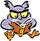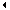HOME / FEEDBACK / ORDER THE BOOK / OTHER BOOKS / THANKS

Certification Practice

Each chapter of the book ends with a Certification Practice question that's comparable to what you could expect to be asked on a Java certification test. Here's the answer for chapter 7:

Answer (c) is correct. The i variable should not be used when calculating the average because it goes up by 1 even when a command-line argument is not a valid floating-point number. For example, if you ran the application like this:

java AverageTest 1 1 1 x

The output would be the following:

Invalid input: x
Average value: 0.75

To correct the problem, the statement should be this:

System.out.println("Average: " + (sum / count));

Answer (a) is incorrect because the statement works correctly. In the conditional part of a for loop, you can put two expressions together with an & operator or one of the other logical operators.

Answer (b) is incorrect because the statement works correctly. Each element in the temps[] array is initialized to 0, so if a NumberFormatException is thrown in the for loop, sum += temps[i] will add 0 to the sum.

Answer (d) is incorrect because there was an error in the application.